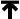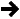Quick Start## Why would you want to use Spirit?

Spirit is designed to be a practical parsing tool. At the very least, the ability to generate a fully-working parser from a formal EBNF specification inlined in C++ significantly reduces development time. While it may be practical to use a full-blown, stand-alone parser such as YACC or ANTLR when we want to develop a computer language such as C or Pascal, it is certainly overkill to bring in the big guns when we wish to write extremely small micro-parsers. At that end of the spectrum, programmers typically approach the job at hand not as a formal parsing task but through ad hoc hacks using primitive tools such as scanf. True, there are tools such as regular-expression libraries (such as boost regex) or scanners (such as boost tokenizer), but these tools do not scale well when we need to write more elaborate parsers. Attempting to write even a moderately-complex parser using these tools leads to code that is hard to understand and maintain.

One prime objective is to make the tool easy to use. When one thinks of a parser generator, the usual reaction is "it must be big and complex with a steep learning curve." Not so. Spirit is designed to be fully scalable. The framework is structured in layers. This permits learning on an as-needed basis, after only learning the minimal core and basic concepts.

For development simplicity and ease in deployment, the entire framework consists of only header files, with no libraries to link against or build. Just put the spirit distribution in your include path, compile and run. Code size? -very tight. In the quick start example that we shall present in a short while, the code size is dominated by the instantiation of the std::vector and std::iostream.

## Trivial Example #1

Create a parser that will parse a floating-point number.

````    `real_p
```

(You've got to admit, that's trivial!) The above code actually generates a Spirit real_parser (a built-in parser) which parses a floating point number. Take note that parsers that are meant to be used directly by the user end with "_p" in their names as a Spirit convention. Spirit has many pre-defined parsers and consistent naming conventions help you keep from going insane!

## Trivial Example #2

Create a parser that will accept a line consisting of two floating-point numbers.

````    ``real_p >> real_p`
```

Here you see the familiar floating-point numeric parser `real_p` used twice, once for each number. What's that >> operator doing in there? Well, they had to be separated by something, and this was chosen as the "followed by" sequence operator. The above program creates a parser from two simpler parsers, glueing them together with the sequence operator. The result is a parser that is a composition of smaller parsers. Whitespace between numbers can implicitly be consumed depending on how the parser is invoked (see below).

Note: when we combine parsers, we end up with a "bigger" parser, But it's still a parser. Parsers can get bigger and bigger, nesting more and more, but whenever you glue two parsers together, you end up with one bigger parser. This is an important concept.

## Trivial Example #3

Create a parser that will accept an arbitrary number of floating-point numbers. (Arbitrary means anything from zero to infinity)

````    ``*real_p`
```

This is like a regular-expression Kleene Star, though the syntax might look a bit odd for a C++ programmer not used to seeing the * operator overloaded like this. Actually, if you know regular expressions it may look odd too since the star is before the expression it modifies. C'est la vie. Blame it on the fact that we must work with the syntax rules of C++.

Any expression that evaluates to a parser may be used with the Kleene Star. Keep in mind, though, that due to C++ operator precedence rules you may need to put the expression in parentheses for complex expressions. The Kleene Star is also known as a Kleene Closure, but we call it the Star in most places.

### Example #4 [ A Just Slightly Less Trivial Example ]

This example will create a parser that accepts a comma-delimited list of numbers and put the numbers in a vector.

#### Step 1. Create the parser

````    ``real_p >> *(ch_p(',') >> real_p)`
```

Notice ch_p(','). It is a literal character parser that can recognize the comma ','. In this case, the Kleene Star is modifying a more complex parser, namely, the one generated by the expression:

````    ``(ch_p(',') >> real_p)`
```

Note that this is a case where the parentheses are necessary. The Kleene star encloses the complete expression above.

#### Step 2. Using a Parser (now that it's created)

Now that we have created a parser, how do we use it? Like the result of any C++ temporary object, we can either store it in a variable, or call functions directly on it.

We'll gloss over some low-level C++ details and just get to the good stuff.

If r is a rule (don't worry about what rules exactly are for now. This will be discussed later. Suffice it to say that the rule is a placeholder variable that can hold a parser), then we store the parser as a rule like this:

````    ``r = real_p >> *(ch_p(',') >> real_p);`
```

Not too exciting, just an assignment like any other C++ expression you've used for years. The cool thing about storing a parser in a rule is this: rules are parsers, and now you can refer to it by name. (In this case the name is r). Notice that this is now a full assignment expression, thus we terminate it with a semicolon, ";".

That's it. We're done with defining the parser. So the next step is now invoking this parser to do its work. There are a couple of ways to do this. For now, we shall use the free parse function that takes in a char const*. The function accepts three arguments:The null-terminated const char* inputThe parser objectAnother parser called the skip parser

In our example, we wish to skip spaces and tabs. Another parser named space_p is included in Spirit's repertoire of predefined parsers. It is a very simple parser that simply recognizes whitespace. We shall use space_p as our skip parser. The skip parser is the one responsible for skipping characters in between parser elements such as the real_p and the ch_p.

Ok, so now let's parse!

````    ````r = real_p >> *(ch_p(',') >> real_p);
parse(str, r, space_p) // Not a full statement yet, patience...```
```

The parse function returns an object (called parse_info) that holds, among other things, the result of the parse. In this example, we need to know:Did the parser successfully recognize the input str?Did the parser fully parse and consume the input up to its end?

To get a complete picture of what we have so far, let us also wrap this parser inside a function:

````    ````bool
parse_numbers(char const* str)
{
return parse(str, real_p >> *(',' >> real_p), space_p).full;
}```
```

Note in this case we dropped the named rule and inlined the parser directly in the call to parse. Upon calling parse, the expression evaluates into a temporary, unnamed parser which is passed into the parse() function, used, and then destroyed.char and wchar_t operands The careful reader may notice that the parser expression has ',' instead of ch_p(',') as the previous examples did. This is ok due to C++ syntax rules of conversion. There are >> operators that are overloaded to accept a char or wchar_t argument on its left or right (but not both). An operator may be overloaded if at least one of its parameters is a user-defined type. In this case, the real_p is the 2nd argument to operator>>, and so the proper overload of >> is used, converting ',' into a character literal parser. The problem with omiting the ch_p call should be obvious: 'a' >> 'b' is not a spirit parser, it is a numeric expression, right-shifting the ASCII (or another encoding) value of 'a' by the ASCII value of 'b'. However, both ch_p('a') >> 'b' and 'a' >> ch_p('b') are Spirit sequence parsers for the letter 'a' followed by 'b'. You'll get used to it, sooner or later.

Take note that the object returned from the parse function has a member called full which returns true if both of our requirements above are met (i.e. the parser fully parsed the input).

#### Step 3. Semantic Actions

Our parser above is really nothing but a recognizer. It answers the question "did the input match our grammar?", but it does not remember any data, nor does it perform any side effects. Remember: we want to put the parsed numbers into a vector. This is done in an action that is linked to a particular parser. For example, whenever we parse a real number, we wish to store the parsed number after a successful match. We now wish to extract information from the parser. Semantic actions do this. Semantic actions may be attached to any point in the grammar specification. These actions are C++ functions or functors that are called whenever a part of the parser successfully recognizes a portion of the input. Say you have a parser P, and a C++ function F, you can make the parser call F whenever it matches an input by attaching F:

````    ``P[&F]`
```

Or if F is a function object (a functor):

````    ``P[F]`
```

The function/functor signature depends on the type of the parser to which it is attached. The parser real_p passes a single argument: the parsed number. Thus, if we were to attach a function F to real_p, we need F to be declared as:

``    ``void F(double n);``

For our example however, again, we can take advantage of some predefined semantic functors and functor generators (A functor generator is a function that returns a functor). For our purpose, Spirit has a functor generator push_back_a(c). In brief, this semantic action, when called, appends the parsed value it receives from the parser it is attached to, to the container c.

Finally, here is our complete comma-separated list parser:

````    ````bool
parse_numbers(char const* str, vector<double>& v)
{
return parse(str,

//  Begin grammar
(
real_p[push_back_a(v)] >> *(',' >> real_p[push_back_a(v)])
)
,
//  End grammar

space_p).full;
}```
```

This is the same parser as above. This time with appropriate semantic actions attached to strategic places to extract the parsed numbers and stuff them in the vector v. The parse_numbers function returns true when successful.The full source code can be viewed here. This is part of the Spirit distribution.